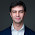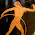## Equivalence Relations

Prerequisites: Sets and Important Notations

Equivalence relations are an important concept that will be needed in some later posts. The good news is that they are relatively easy to understand.

For the rest of this post, let $A$ be a set.

A binary relation on $A$ is a set $R$ of ordered pairs of elements of $A$, i.e. $\{(x,y) \in A \times A \ \colon \ ...{\scr some~condition~on~}x {\scr~and~}y \}$ ($A \times A$ is the set of ordered pairs of elements of $A$). If $(x,y) \in R$, we write $x \sim y$ or sometimes $x \equiv y$. Confused? It's easier to understand with an example- I'll present two, one of which will turn out to be an equivalence relation while the other will not.

Let $P$ be the set of all living people on earth and for $x,y \in P$, say $x \sim y$ if $x$ and $y$ have the same birthday. This will turn out to be an equivalence relation on $P$.

Here's another example that is not an equivalence relation (you'll see why below). Let $\Bbb R$ be the set of real numbers, i.e. the number line. Don't worry about the precise definition- there will be a few posts dedicated to that later. For now, just think of $\Bbb R$ as the number line which we all know and love since first grade. Then $\leq$ is a binary relation on $\Bbb R$. If we wanted to use the set formulation above, we could call the relation $L = \{ (x,y) \in {\Bbb R} \times {\Bbb R} \ \colon \ x \leq y \}$. Note that the order of $x$ and $y$ clearly matter here, since $5 \leq 7$, but $7 \nleq 5$. So it's important that a binary relation is a set of ordered pairs of elements of our set.

Now for the main event.

An equivalence relation on $A$ is a binary relation $\sim$ on $A$ that satisfies the following three properties for any $x,y,z \in A$:
(1) $x \sim x$ (reflexivity)
(2) If $x \sim y$, then $y \sim x$ as well (symmetry), and
(3) If $x \sim y$ and $y \sim z$, then $x \sim z$ (transitivity).

The birthday relation above clearly satisfies these properties and is thus an equivalence relation on the set $P$ of people. The relation $\leq$ on $\Bbb R$ is not an equivalence relation because, while it does satisfy (1) and (3), it does not satisfy (2), since $5 \leq 7$, but $7 \nleq 5$.

An equivalence relation partitions the set into equivalence classes. For $x \in A$, the equivalence class of $x$, denoted $[x]$, is defined as the set of elements of $A$ that are equivalent to $x$, i.e. $[x] = \{ y \in A~\colon~y \sim x \}$.

The set of all equivalence classes of $A$ under $\sim$ is called the quotient set of $A$ by $\sim$ and is denoted $A/{\sim}$. $A$ is indeed partitioned by $\sim$ in the sense that:
$$\biguplus_{C \in A/{\sim}} C = A$$
This means that, first of all, the equivalence classes $[x]$ (remember, the $C$'s above are elements of $A/{\sim}$, i.e. equivalence classes $[x]$) cover all of $A$ (that is, $\forall~y \in A,~\exists~x \in A {\scr~such~that~} y \in [x]$. In fact, this $x$ is just $y$ itself, because $y$ is always in $[y]$. Can you see why from the definition of equivalence class?). Notice the little $+$ inside the union symbol? This is the other part of partition thing, and it means that the sets being "unioned" are disjoint- they do not have any overlap. To be precise, "no overlap" means that their intersection is empty: $\forall~x,y, \in A$ with $x \nsim y$, $[x] \cap [y] = \emptyset$.

So the equivalence classes (this is, the elements of the set $A/{\sim}$) cover all of $A$ in the sense that each element of $A$ is in one of them, and they do not overlap. We can actually prove the latter fact using the definition of an equivalence relation:

Let $x,y \in A$ with $x \nsim y$, and assume that $[x] \cap [y] \neq \emptyset$, i.e. $\exists~z \in A$ such that $z \in [x] \cap [y]$ (i.e. $z$ is in both $[x]$ and $[y]$). We are going to prove that this leads to a contradiction. $z \in [x]$ means that $z \sim x$. The symmetry property (2) tells us it's also true that $x \sim z$. Similarly, $z \in [y]$ means that $z \sim y$. Since $x \sim z$ and $z \sim y$, the transitive property (3) of equivalence relations implies that $x \sim y$. But that contradicts the assumption that $x \nsim y$. Thus, there cannot exist such a $z$, and the equivalence classes $[x]$ and $[y]$ do not overlap. So it was legit for us to use the $\biguplus$ symbol above, and an equivalence relation indeed constitutes a partition of $A$.

In fact, any partition also defines an equivalence relation. Suppose we have a collection $\{X_i\}_{i \in I}$ of subsets of $A$, indexed by some index set $I$ (an index set is just a set of labels for a collection of other things, in this case subsets of $A$- usually you use one when you aren't sure whether there will be a countable or uncountable number of items in your collection that need a label, in which case you can't just number the items) such that $A = \biguplus_{i \in I} X_i$. Then the $X_i$'s define an obvious equivalence relation on $A$ by $x \sim y$ if there exists a single $i \in I$ such that $x,y \in X_i$.

Sorry that this post is not that exciting, but equivalence relations are an important concept which I promise will be used in at least one cool post later. To liven things up a bit, there is a surprise, and that is that during the discussion above, we actually proved the so-called Fundamental Theorem of Equivalence Relations, which states that given a set $A$ and an equivalence relation $\sim$ on $A$,
(1) $\sim$ partitions $A$ in the sense described above, and
(2) every partition of $A$ defines an equivalence relation on $A$.

Thanks for reading, and feel free to post any questions in the comments section.

1.Complex, But thou made it simple.. by stopping at the right point for starters:)
Equivalence, Partition & Disjoint lead to such a cool logic in Lattice Theory; I love Equivalence Relations!

Some other examples of Equivalence
*Set of numbers having the same parity - even/odd
*Set of roommates (A sharing room with B, B sharing room with C)

Question:
Is Equality, an Equivalence Relation?
Everyone getting the same bonus, 50k=50k=50k :))

Tricky Question:
Is Harry Potter, friend of Hermoine, friend of Ron Weasley - an equivalence relation?
Hope u welcome harry potter questions, lol:)

1.Equality of numbers is an equivalence relation because $\forall~x,y,z \in {\Bbb R}$, we have $x=x$, $x=y$ if and only if $y=x$, and if $x=y$ and $y=z$, then $x=z$. In fact, equality is the finest equivalence relation on ${\Bbb R}$ in the sense that it produces the smallest possible equivalence classes. In particular, each equivalence class is a single number, i.e. a single element of ${\Bbb R}$.

Friendship is not an equivalence relation because, even if we assume it's symmetric, it's not transitive: if Harry is friends with Hermione, and Hermione is friends with Ron, that does not necessarily mean that Harry is friends with Ron. Also, Harry may consider himself his own friend, but if he does, that's pretty sad...

2.//Hope u welcome harry potter questions, lol:)//

Omg! See who is this?:) What has dumb Harry Potter to do with a great subject like math? lol.
Anyways, Harry never figures out anything great, It's all the bookish Hermione who can take a math credit:)

2.Good Blog; Can be a bit more simple though.
Of all the postings, I feel this one is the most simple.

We all kind of develop a feeling that math is throttled down our throats in the college, because of absence of real life examples or relating the concepts to day to day life. For that matter, I like math when it is combined with physics.
Hope such blogs can help overcome that math phobia, that most of us have with real life examples. Wish you all the success.

Just checking some questions. Is the set of all road runner bikes with the same colour, an equivalence class?
Or set of students in the class, having the same age or same hobby, or even same crush on X? lol.